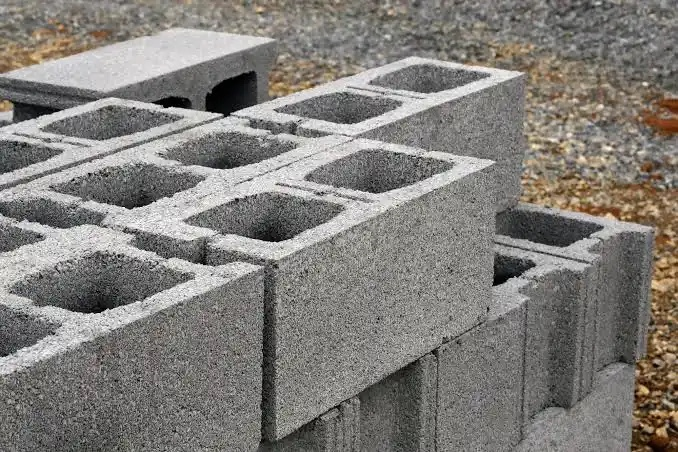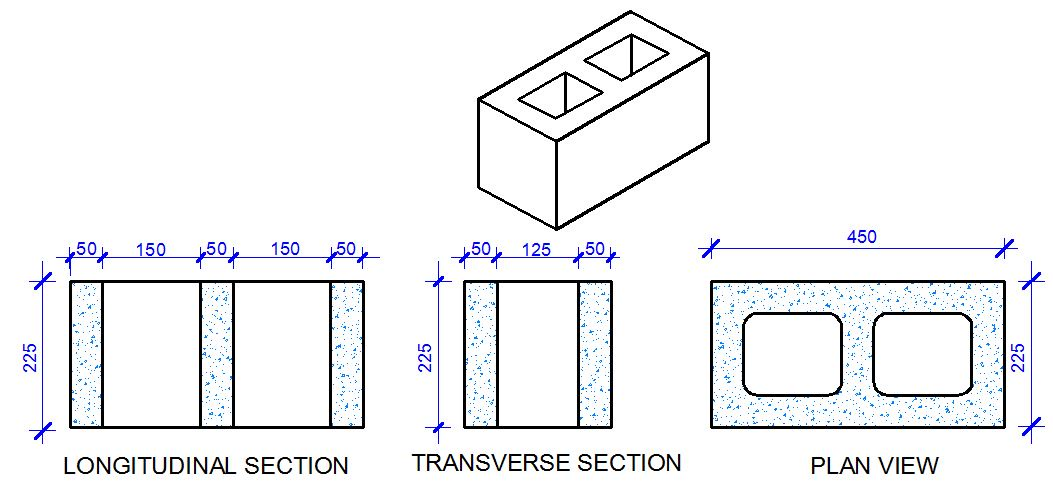Sign in#In Nigeria, the 9 inches hollow block (450mm x 225mm x 225mm) is normally used for the construction of blockwork of buildings exceeding one storey. The length of the block is 450mm (18 inches), the height is 225mm (9 inches), and the width is 225mm (9 inches). Contractors and builders are often faced with the challenge of either buying blocks for construction or moulding

it on their own. When the conditions are right, builders will prefer to mould their own blocks, especially for the purpose of controlling the quality of the blocks, and to reduce the cost of transportation.

Let us assume that you have decided to mould your own blocks, and you have been faced with the challenge of estimating the quantity of cement and sand to purchase that will satisfy the construction requirement.

This article will give you a guide on how to estimate the quantity of sand and cement required for moulding blocks in Nigeria. I am going to work through the steps, so that you will be able to make calculations just in case you are using any other size of block.

Initial data

Number of blocks required = 3000 pieces

Recommended production = 1 bag of cement to produce 35 blocks

Step 1: Calculate the volume of the blockFor the block size shown above;

Volume of block without holes = (0.45 × 0.225 × 0.225) = 0.02278125 m3

Volume of holes = 2(0.225 × 0.125 × 0.15) = 0.0084375 m3

Therefore volume of the block = 0.02278 – 0.0084375 = 0.0143 m3

Step 2: Calculate the volume of the 35 blocks

If the volume of 1 block is 0.0143, the volume of 35 blocks = (0.0143 × 35) = 0.502 m3

Step 3: Calculate the volume sand required for the 35 blocks

The volume of 1 bag of cement is 34.72 litres = 0.03472m3.

This is obtained by knowing that the mass of 1 bag of cement = 50kg, and the density = 1440 kg/m3

The volume of a standard builder’s wheelbarrow is 0.065 m3 (unheaped). We assume that approximately 2 bags of cement (4 head pans of cement) will fill one builder’s wheelbarrow. Now, we can estimate the number of wheelbarrow trips of sand that the moulder should provide in order to make 35 blocks from one bag of cement.

Total volume of 35 blocks required = 0.502 m3, Let the number of wheel barrow trips of sand be x

Hence, (volume of 1 bag of cement) + (Total volume of sand) = Volume of 35 blocks

Hence, 0.03472 + x(0.065) = 0.502 m3

On solving, x = 7.1889 unheaped wheelbarrow trips of sand

The volume of sand required to make 35 blocks = 7.1889 × 0.065 = 0.46728 m3

However, note that this leads to a mix ratio of about 1:14 which will not be very good when subjected to a compressive strength test. The ideal mix ratio for blocks for proper strength should be about 1:10 or 1 bag to 25 blocks.

Step 4: Calculate the total volume of materials required

We can therefore estimate the quantity of materials to be purchased;

If 1 bag of cement is needed for 35 blocks, therefore 86 bags of cement is needed to mould 3000 blocks (gotten by 3000/35)

If 0.46728 m3 of sand is required to make 35 blocks, therefore, 41 m3 of sand (about 67.60 tonnes assuming the density of dry sand = 1600 kg/m3) is needed to make 3000 blocks.

For a 5 tonne tipper of 3.8 m3 capacity, we have to order for 11 trips of sharp sand.

You have to be very sure of the size of the tipper that will be delivering the sand to you in order to make your estimates more reasonable. On the other hand, you can make the considerations for variations between the weight of materials when wet and when dry. However, this will never be critical for block moulding based on experience. But in concrete it can be very considerable.

Therefore summarily, we need 86 bags of cement and 68 tonnes of sand to mould about 3000 pieces of 9 inches blocks with holes (for 1 bag = 35 blocks).

Content created and supplied by: ewhomas24 (via Opera News )

Nigeria